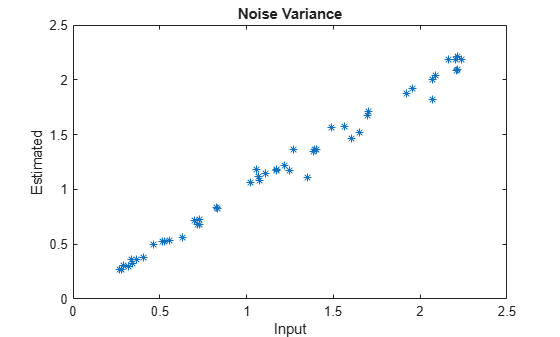# arburg

Autoregressive all-pole model parameters — Burg’s method

## Syntax

``a = arburg(x,p)``
``[a,e,rc] = arburg(x,p)``

## Description

example

````a = arburg(x,p)` returns the normalized autoregressive (AR) parameters corresponding to a model of order `p` for the input array `x`.```
````[a,e,rc] = arburg(x,p)` also returns the estimated variance, `e`, of the white noise input and the reflection coefficients, `rc`.```

## Examples

collapse all

Use a vector of polynomial coefficients to generate an AR(4) process by filtering 1024 samples of white noise. Reset the random number generator for reproducible results. Use Burg's method to estimate the coefficients.

```rng default A = [1 -2.7607 3.8106 -2.6535 0.9238]; y = filter(1,A,0.2*randn(1024,1)); arcoeffs = arburg(y,4)```
```arcoeffs = 1×5 1.0000 -2.7743 3.8408 -2.6843 0.9360 ```

Generate 50 realizations of the process, changing each time the variance of the input noise. Compare the Burg-estimated variances to the actual values.

```nrealiz = 50; noisestdz = rand(1,nrealiz)+0.5; randnoise = randn(1024,nrealiz); noisevar = zeros(1,nrealiz); for k = 1:nrealiz y = filter(1,A,noisestdz(k) * randnoise(:,k)); [arcoeffs,noisevar(k)] = arburg(y,4); end plot(noisestdz.^2,noisevar,'*') title('Noise Variance') xlabel('Input') ylabel('Estimated')```Repeat the procedure using the function's multichannel syntax.

```Y = filter(1,A,noisestdz.*randnoise); [coeffs,variances] = arburg(Y,4); hold on plot(noisestdz.^2,variances,'o') hold off legend('Single channel loop','Multichannel','Location','best')```## Input Arguments

collapse all

Input array, specified as a vector or matrix.

Example: `filter(1,[1 -0.75 0.5],0.2*randn(1024,1))` specifies a second-order autoregressive process.

Data Types: `single` | `double`
Complex Number Support: Yes

Model order, specified as a positive integer scalar. `p` must be less than the number of elements or rows of `x`.

Data Types: `single` | `double`

## Output Arguments

collapse all

Normalized autoregressive parameters, returned as a vector or matrix. If `x` is a matrix, then each row of `a` corresponds to a column of `x`. `a` has `p` + 1 columns and contains the AR system parameters, A(z), in descending powers of z.

White noise input variance, returned as a scalar or row vector. If `x` is a matrix, then each element of `e` corresponds to a column of `x`.

Reflection coefficients, returned as a column vector or matrix. If `x` is a matrix, then each column of `rc` corresponds to a column of `x`. `rc` has `p` rows.

collapse all

### AR(p) Model

In an AR model of order p, the current output is a linear combination of the past p outputs plus a white noise input.

The weights on the p past outputs minimize the mean squared prediction error of the autoregression. If y(n) is the current value of the output and x(n) is a zero mean white noise input, the AR(p) model is:

`$y\left(n\right)+\sum _{k=1}^{p}a\left(k\right)y\left(n-k\right)=x\left(n\right).$`

### Reflection Coefficients

The reflection coefficients are the partial autocorrelation coefficients scaled by –1. The reflection coefficients indicate the time dependence between y(n) and y(n – k) after subtracting the prediction based on the intervening k – 1 time steps.

## Algorithms

Burg's method estimates the reflection coefficients and uses the reflection coefficients to estimate the AR parameters recursively. You can find the recursion and lattice filter relations describing the update of the forward and backward prediction errors in .

 Kay, Steven M. Modern Spectral Estimation: Theory and Application. Englewood Cliffs, NJ: Prentice Hall, 1988.